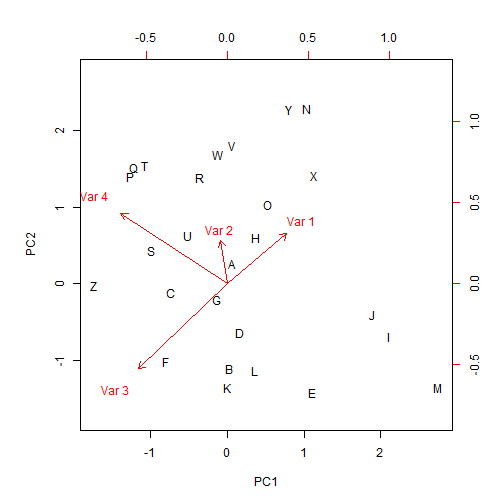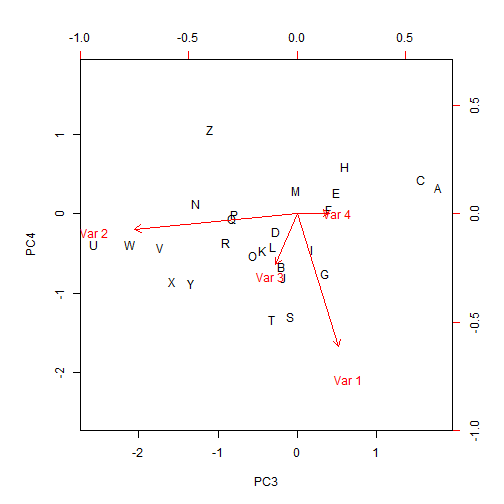## Monday, March 30, 2015

### Phylogenetic PCA 'biplot' with choices argument

A phytools user recently asked the following:

“I am trying to plot the results of a phylogenetic PCA generated with your package Phytools. I would especially like to plot the third and fourth components using the "choices” option of the `biplot()` function in R. My feeling is that this option is not implemented while using your `phyl.pca` function since I get the message `"choices" is not a graphical parameter`. Am I right? Is there a way I can plot other components than the default ones?“

In fact, this is correct. Here is the function code for `biplot.phyl.pca` (originally suggested to me by Joan Maspons):

``````library(phytools)
biplot.phyl.pca
``````
``````## function (x, ...)
## {
##     biplot(x\$S, x\$L, ...)
## }
## <environment: namespace:phytools>
``````
``````tree<-pbtree(n=26,tip.label=LETTERS)
X<-fastBM(tree,nsim=4)
obj<-phyl.pca(tree,X)
obj
``````
``````## Phylogenetic pca
## Starndard deviations:
##       PC1       PC2       PC3       PC4
## 1.0959134 0.9057384 0.8286637 0.6021671
##              PC1        PC2        PC3          PC4
## [1,]  0.45746491  0.3875983  0.2376398 -0.764212515
## [2,] -0.05002709  0.3353159 -0.9366821 -0.087676734
## [3,] -0.68744007 -0.6538311 -0.1233330 -0.291066912
## [4,] -0.81927353  0.5421294  0.1867339  0.004131128
``````
``````biplot(obj)
````````````biplot(obj,choices=c(3,4)) ## doesn't work
``````
``````## Warning in plot.window(...): "choices" is not a graphical parameter
``````
``````## Warning in plot.xy(xy, type, ...): "choices" is not a graphical parameter
``````
``````## Warning in axis(side = side, at = at, labels = labels, ...): "choices" is
## not a graphical parameter
``````
``````## Warning in axis(side = side, at = at, labels = labels, ...): "choices" is
## not a graphical parameter
``````
``````## Warning in box(...): "choices" is not a graphical parameter
``````
``````## Warning in title(...): "choices" is not a graphical parameter
``````
``````## Warning in text.default(x, xlabs, cex = cex[1L], col = col[1L], ...):
## "choices" is not a graphical parameter
``````
``````## Warning in plot.window(...): "choices" is not a graphical parameter
``````
``````## Warning in plot.xy(xy, type, ...): "choices" is not a graphical parameter
``````
``````## Warning in title(...): "choices" is not a graphical parameter
``````
``````## Warning in axis(3, col = col[2L], ...): "choices" is not a graphical
## parameter
``````
``````## Warning in axis(4, col = col[2L], ...): "choices" is not a graphical
## parameter
``````
``````## Warning in text.default(y, labels = ylabs, cex = cex[2L], col = col[2L], :
## "choices" is not a graphical parameter
``````It is non-trivial, but fairly straightforward, to modify this to permit use of the argument `choices` to select PC axes to be plotted. For this I made use of the `do.call` function:

``````biplot.phyl.pca<-function(x,...){
to.do<-list(...)
if(hasArg(choices)){
choices<-list(...)\$choices
to.do\$choices<-NULL
} else choices<-c(1,2)
to.do\$x<-x\$S[,choices]
to.do\$y<-x\$L[,choices]
do.call(biplot,to.do)
}
``````

Let's try it:

``````biplot(obj) ## standard biplot
````````````biplot(obj,choices=c(3,4))
``````That's it. Assuming I don't find (and no one points out) an error in this, I will add this to the next version of phytools.

1.Hi Liam,

Thanks for these usefull package and blog !

Currently Master in France, Montpellier, I would like to use the phyl.pca funtion to select the most important climatic variables (starting with the 19 bioclim variables).
I have the result\$L giving the variables position for each of PC. Do you have any clues which can help me to obtain the variable contributions ? The FactoMineR do it with the function 'fviz_contrib' but I cant use the result\$L as a imput.

Best regards

Pierre

1.Hi again Liam,
I reword my question : Do you have any clue to find the cos 2 values ?